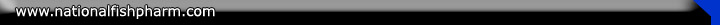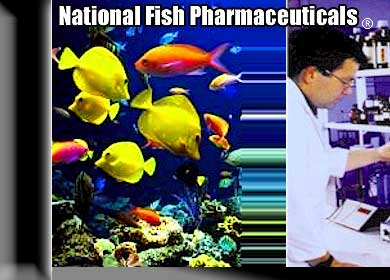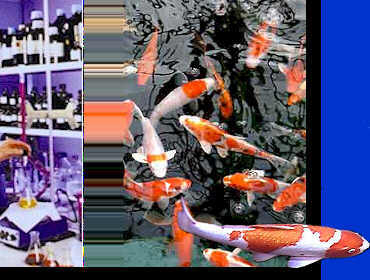# Water Volume Calculation & Conversion Charts

Calculating Water Volume In U.S. Gallons

1.  Mathematical Formulas

a.  If computed in inches, divide the total by 231.
b.  If computed in feet, multiply the total by 7.5
Rectangular shapes: length X width X depth.
Circular shapes: 3.14 X radius² X depth

2.  Using a domestic water meter:  Use a wrench to turn the locking nut on the meter to remove the lid.  Record the reading before filling the pond.  Subtract this figure from the figure recorded after filling is completed.  No water at all should be used in the house during filling.

3.  Using hose output:  Fill a large bucket for exactly 60 seconds.  Measure the water in pints and divide by 8 to compute U.S. gallons.  Record start and stop times of filling the pond.  Multiply the total minutes required for filling by the number of gallons the hose discharges in one minute.

Calculating Water Volume In Liters

1.  Multiply length X width X depth in meters X 28.41 for rectangular volume.

2.  Multiply depth in meters X metric diameter² X 20.75 for circular volume.

 Frequently Used Equivalents ppm (parts per million) is equivalent to one milligram per liter of water. 5ml = 1 teaspoon 20 drops = 1 ml. 60 drops = 1 teaspoon 15ml = 1 tablespoon 2 tsp. = 1 dessert spoon 2 dessert spoons = 1 tablespoon 2 tbsp. = 1 fl. oz. 8 fl. oz. = 1 cup 1 cup = 48 teaspoons 1 cup = 16 tablespoons 1 cup = 237ml. *These figures are commonly supplied by most chart sources.  However, using a standard, pharmacist-supplied, two-milliliter eye dropper and a standard kitchen measuring teaspoon, the equivalency was found to be 3ml/tsp. and 9ml/tbsp.  *Culinary, or food industry measuring devices and weights are different from pharmaceutical and laboratory measuring devices. Table of Liquid Equivalents 1 milliliter (ml) = 1 cm³ = 1cc = 20 drops = 0.20 tsp. = 0.061 in.³ = 0.001 L = 1gm. of water = 0.002 lb. of water = 0.0003 U.S. gallons 1 U.S. Gallon (gal.) = 3.785 L = 0.1339 ft.³ = 231 in.³ = 8.345 lb. of water = 3785.4 gm. of water = 4qt. = 8 pt. = 135.52 oz. = 128 fluid oz. = 3785.4 ml 1 fluid ounce = 6 tsp. = 2 tbsp. = 0.0078 U.S. gal. = 0.031 qt. = 29.57 gm. = 0.062 pt. = 0.065 lb. = 1.04 oz. 1 Imperial gallon = 4.5459L = 0.1605 ft.³ = 277.42 in. ³ 4.845 qt.
 Weight Equivalents Parts Per Million 1 grain (gr.) = 64.8 mg. = 0.065 gm.= 0.35 oz.1 gram (gm.) = 15.432 gr.= 0.0353 fl. oz. = 0.0022 lb.= 0.002 pt.= 0.001 L = 1000 mg.= 0.001 kg.1 ounce (oz.) = 480 gr.= 28.35 gm.= 0.0075 gal.= 0.03 qt.= 0.06 pt. = 0.0625 lb.=0.96 fl. oz. 1 pound (lb.) = 5760 gr. = 373.24 gm. 1 pound, avoirdupois = 7000 gr. = 453.6 grams = 16 oz. = 0.12 gal = 0.016 ft.³ of water = 0.48 qt. = 0.96 pt. = 15.35 fl. oz = 458.59 cc or cm³ = 453.59 ml. = 453.59 gm. = 0.454 L Leave this text in this area for sizing purposes 1 ppm = 1 mg/L= 3.8 mg/gal. = 2.7 lb./acre foot= 0.0038 gm/gal. = 0.0283 gm/ft.³ 1 oz./1000 ft.³ = 0.0000623 lb./ft.³ = 0.0586 gr./gal. = 8.34 lb./million gallons water = 0.134 oz./1000 gal. = 1 oz./1000 ft.³ water = 1 gm./264 gal. water = 1 gm./m³ water 1 gr./gal. = 19.12 ppm.
©1995-2006 www.nationalfishpharm.com - Site Map - Site Design by Brian Aukes & AFAM LLC.